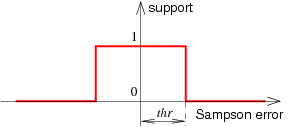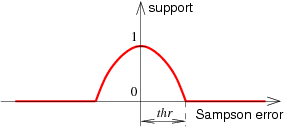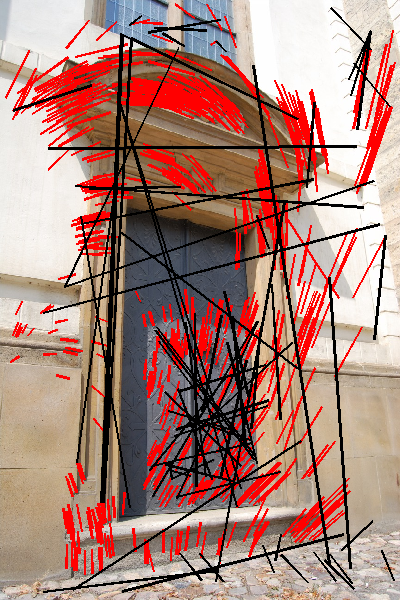Warning

# Robust Estimation of Calibrated Epipolar Geometry of Image Pairs

Calibrated epipolar geometry is characterised by essential matrix, that encodes relative translation and rotation. The matrix can be estimated using image-to-image correspondences. Since the tentative correspondences are assumed to contain errors, a robust estimation method should be used. Then an epipolar geometry is found, together with a sub-set of correspondences that are consistent with it (allowing some defined inaccuracy). One possible method is the RANSAC scheme: hypothesis of essential matrix is generated and its consistence with data (correspondences) is verified. These two steps are repeated and the hypothesis most consistent with data is chosen.

#### Hypothesis Generation

The images are captured by a calibrated camera (K is known), so the corresponding image points are first transformed by. Then the essential matrix E is estimated. This matrix has five degrees of freedom and can be computed from five point correspondences. The five-point algorithm is available in code repository (package p5). Then the hypothesis generation can be summarised by following procedure:

1. Chose a random 5-tuple of correspondences (points u1 in the first image and u2 in the second one).
2. Compute essential matrices E from u1 a u2. There can be more than one solution given by the five-point algorithm typically. Each one must be treated independently.
3. Decompose every E into rotations R and translations t - four combinations. The rotation and translation can be used to construct a camera pair and to reconstruct the 3D positions of the five corresponding points. Select such R, t from the four combinations for which the five reconstructed 3D points lie in front of both cameras. For a particular E there can be no solution as well.

In order to test that the 3D points are in front of cameras, the cameras necessary for triangulation of u1 and u2 must be constructed. Since the points have allready K undone, the cameras will be P1 = [I|0] (canonical one) and P2 = [R|t].

#### Verification of Consensus of Hypothesis and Data

Every pair of R, t must be verified against all correspondences. Given some robust function, a measure of consensus (a support) is computed as a sum of contributions of all single correspondences. A reprojection eroor (using e.g. Sampson approximation) must be estimated for each correspondence. A contribution of every single correspondence is then computed from this error by chosen robust function. In practice, we can use (figure 1):

1. thresholding: if the error is lower than threshold, count 1; otherwise count 0
2. approximation of ML estimator: if the erroris lower than threshold, count, otherwise count 0.Fig. 1: Contribution of a single correspondence: thresholding (left) and ML approximation (right).

The correspondences with reprojection error below the threshold are assumed correct (inliers), the others are assumed incorrect (outliers). The correspondences with error below the threshold must be again verified if they lead to points in front of both cameras (e.g. by triangulation), and the bad ones must be considered as outliers and its support counted as 0.

As a result of robust estimation, there is epipolar geometry characterised by rotation R and translation t and separation of correspondences into inliers and outliers, figure 2.Fig. 2: Inlier (červeně) and outlier (černě) correspondences, shown in the first image as lines connecting corresponding points from the first and from the second image.